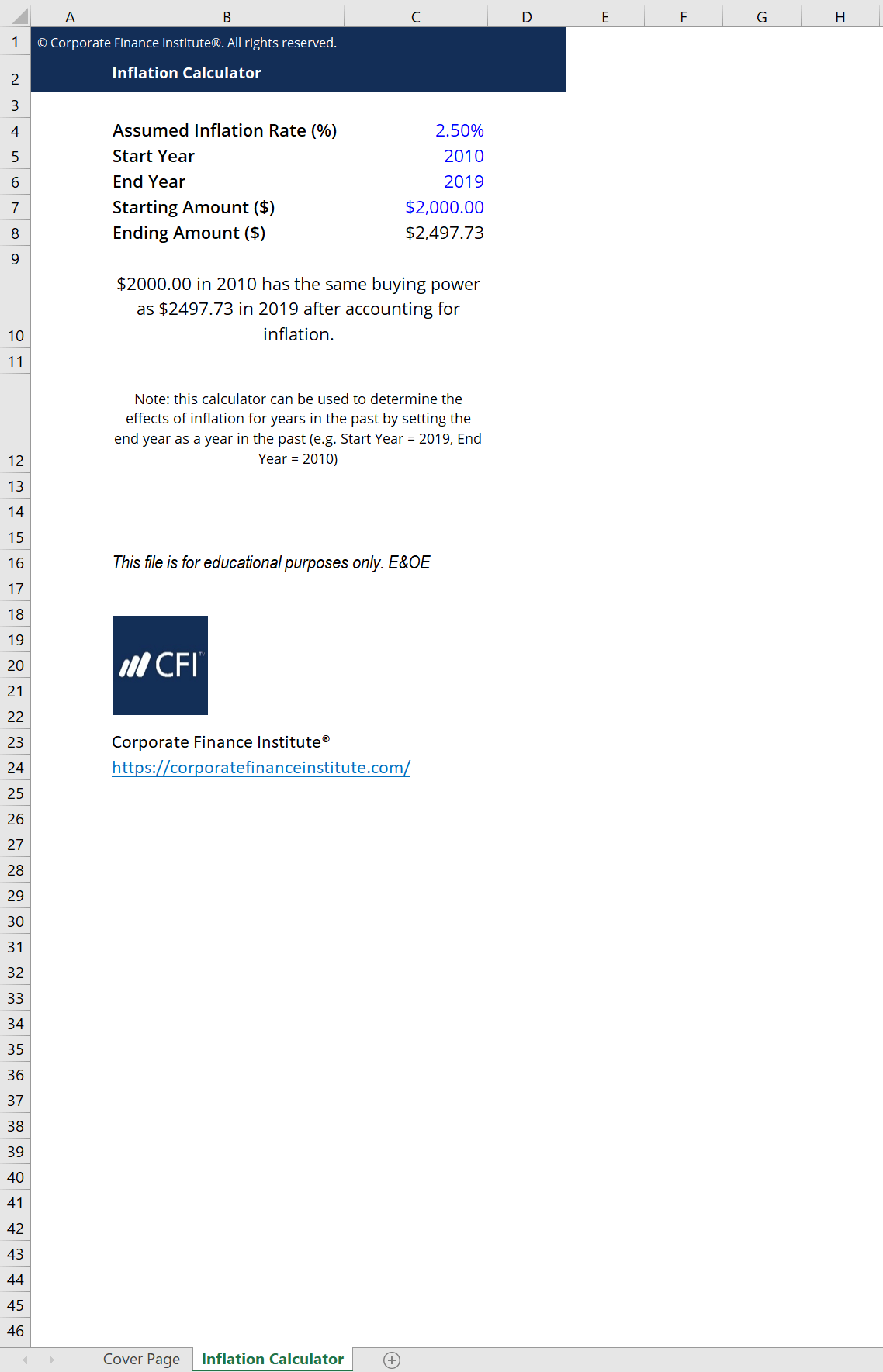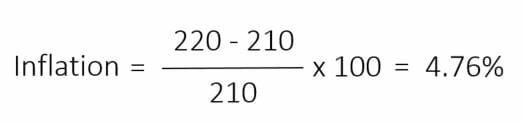# Inflation Calculator

## Inflation Calculator

This inflation calculator will help you figure out how much your money will be worth after a period of time as a result of inflation – the increase in the price level of goods over time.

Check out the inflation calculator preview below:### Calculating Inflation Rates

Inflation is an economic concept pertaining to how prices of goods increase over a period of time. Inflation rates are typically calculated by observing changing in price indices. Usually, changes in the Consumer Price Index (CPI) are used as the basis for inflation. Consider the following example, the CPI for Country A was 210 at 2017 year end (base year). At 2018 year end, the CPI was 220. By calculating the percent change, we can see the inflation over this time period:Once the inflation rate is calculated, you can input it into CFI’s inflation calculator to see its effect on the value of money over time.

### Effect of Inflation

The overall rise in price level means that money in that economy loses purchasing power. In other words, having \$100 today is worth more than having \$100 in 10 years, due to natural inflationary effects.

We can look at the inflation calculator preview above for an example. In the preview, the assumed inflation rate is 2.5%. That means that every year, the general price level of goods in the economy increases by 2.5%. Therefore, your money needs to keep up and increase by at least 2.5% to stay equal to the economy. Thus, if you were to compare \$2,000.00 in 2010, it would increase by 2.5% each year for 9 years to reach \$2,497.73 by 2019.

### More Free Templates

For more resources, check out our business templates library to download numerous free Excel modeling, PowerPoint presentation and Word document templates.

• Excel Modeling Templates
• PowerPoint Presentation Templates
• Transaction Document Templates
• CFI Template Marketplace

### Financial Analyst Certification

Become a certified Financial Modeling and Valuation Analyst (FMVA)® by completing CFI’s online financial modeling classes and training program!